# Make Genome Level Trellis Graph

Author: Zuguang Gu ( z.gu@dkfz.de )

Date: 2015-04-16

Trellis graph is a type of graph which splits data by certain conditions and visualizes subset of data in each category parallel. The advantage of Trellis graph is that it can easily reveal multiple variable relationship behind the data. For genomic data, chromosomes are always the category variable. In R, lattice and ggplot2 package can make Trellis graph, however, specially for whole genome level plot, they are limited in:

• Chromosomes have different length. But in lattice/ggplot2, panel width for each chromosome are the same, so short chromosomes will be extended with empty areas which sometimes is a waste of space.
• Genomic data are more multiple dimensional, which means, there are always more than one tracks for chromosomes that to be compared. Unfortunately, lattice/ggplot2 can only plot one track at one time.

For single continuous region, multiple tracks are supported in ggbio and Gviz. But if you want to compare more than one continuous regions, things will be complex. Anyway, you can use `grid.layout` to arrange multiple continuous regions on the plot with using ggbio and Gviz, but the solution is not so straightforward.

Here, gtrellis provides a flexible way to arrange genomic categories and supports adding self-defined graphics on the plot.

## Basic design

gtrellis aims to arrange genomic categories as Trellis style and supports multiple tracks for visualization. In this package, initialization the layout and adding graphics are independent. After initialization of the layout, intersection between tracks and genomic categories are named cell or panel, and each cell is an independent plotting region (actually, each cell is a data viewport in grid system) that self-defined graphics can be added afterwards.

gtrellis is implemented in grid graphic system, so, in order to add graphics in each cell, you only need to use low-level graphic functions (`grid.points`, `grid.lines`, `grid.rect`, …) which are quite similar as those in classic graphic system. There is only one thing you need to take care of is the use of `unit` object in grid system.

## Initialize the layout

`gtrellis_layout()` is used to create the global layout. By default, it initializes the layout with hg19 and puts all chromosomes in one row. Each chromosome has only one track and range on y-axis is 0 to 1.

``````library(gtrellis)
gtrellis_layout()
``````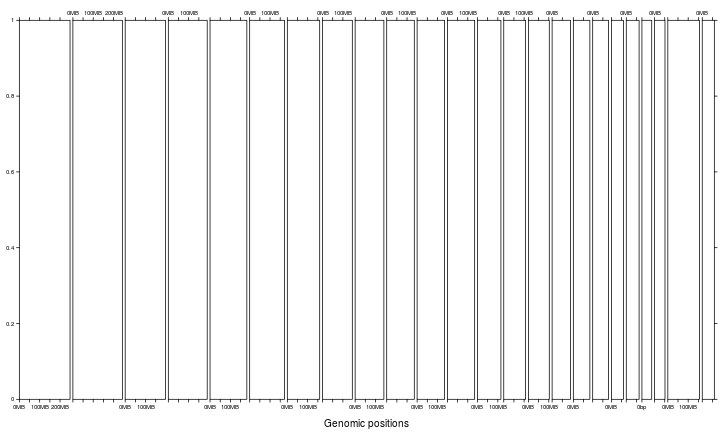`category` can be used to set subset of chromosomes as well as the order of chromosomes. `gtrellis_show_index()` here is an assistant function to add the information to each cell.

``````gtrellis_layout(category = c("chr3", "chr1"))
gtrellis_show_index()
``````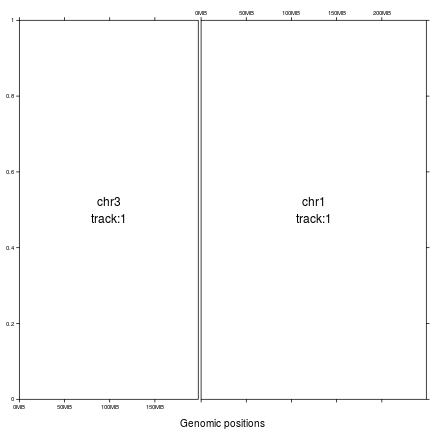Other species are also supported as long as corresponding chromInfo files exist on UCSC ftp. E.g. chromInfo file for mouse (mm10) is http://hgdownload.cse.ucsc.edu/goldenpath/mm10/database/chromInfo.txt.gz. Since there may be many short scaffolds in chromInfo file, `gtrellis` will first remove these short scaffolds before making the plot.

``````gtrellis_layout(species = "mm10")
gtrellis_show_index()
``````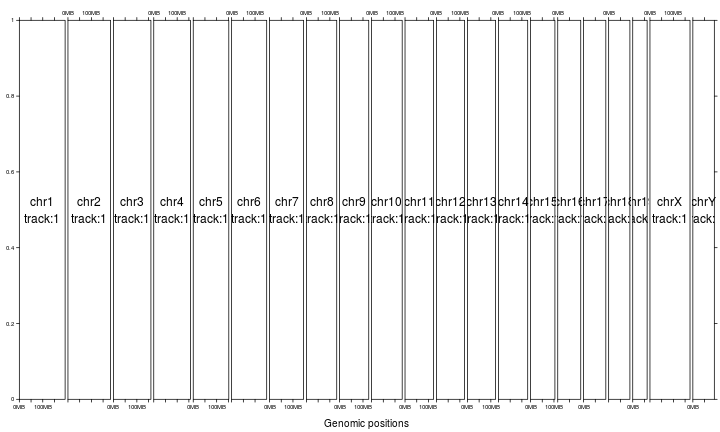You can put chromosomes on multiple rows by specifying `nrow` or/and `ncol` in case you feel the width for some chromosomes are too short. For chromosomes in the same column, the corresponding width is the width of the longest chromosome in that column and short chromosomes will be extended with empty areas.

``````gtrellis_layout(nrow = 3)
gtrellis_show_index()
``````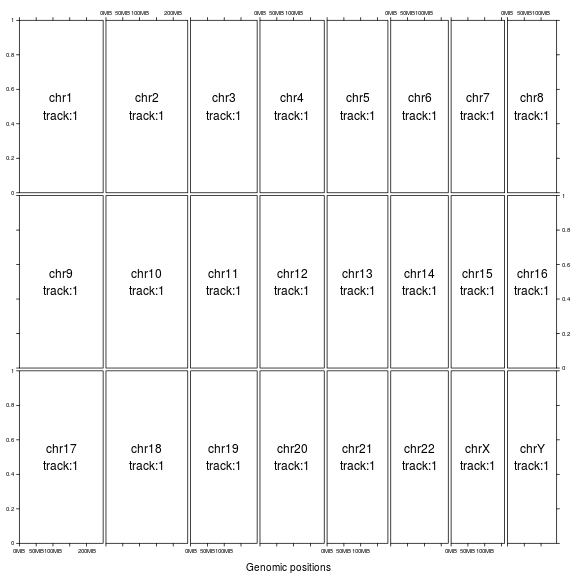``````gtrellis_layout(ncol = 5)
gtrellis_show_index()
``````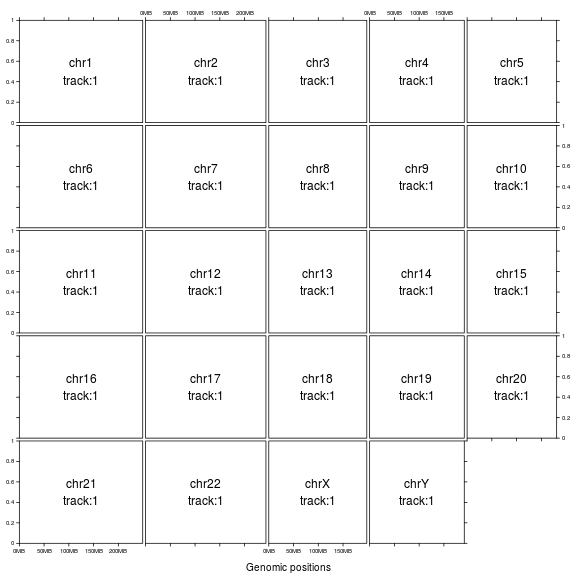You can set `byrow` argument to arrange chromosomes either by rows or by columns. As explained before, by default chromosomes in the same column will share the length of the longest one. It is better to put chromosomes with similar length in a same column.

``````gtrellis_layout(ncol = 5, byrow = FALSE)
gtrellis_show_index()
``````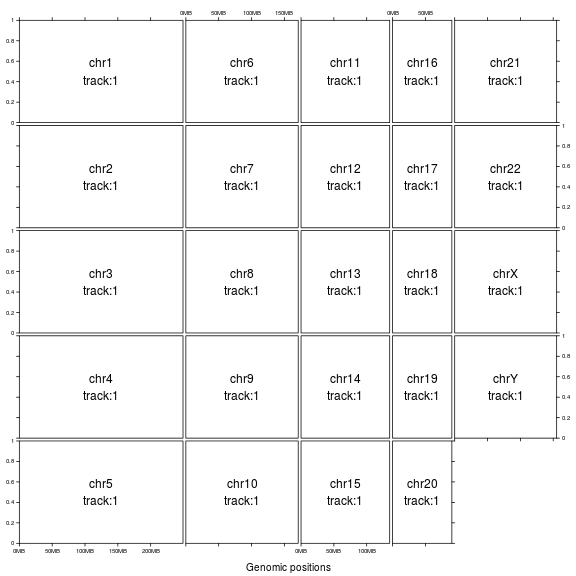If `equal_width` is set to `TRUE`, the layout will be a 'standard' Trellis layout. All chromosomes will share the same range on x-axis (length of the longest chromosome) and short chromosomes will be extended with empty areas.

``````gtrellis_layout(equal_width = TRUE)
gtrellis_show_index()
``````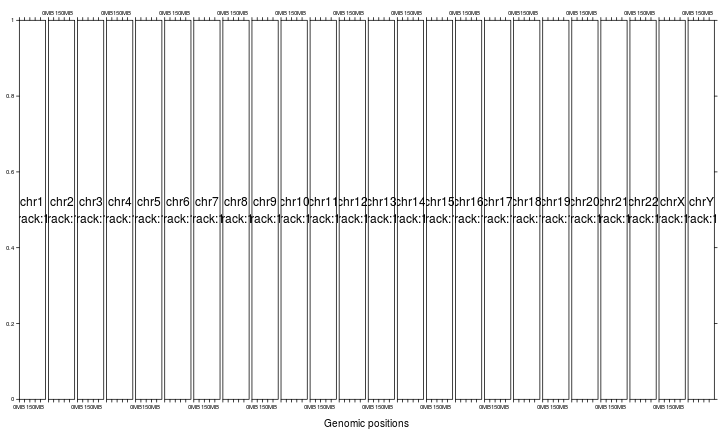Make all columns having equal width and also set multiple rows.

``````gtrellis_layout(ncol = 5, byrow = FALSE, equal_width = TRUE)
gtrellis_show_index()
``````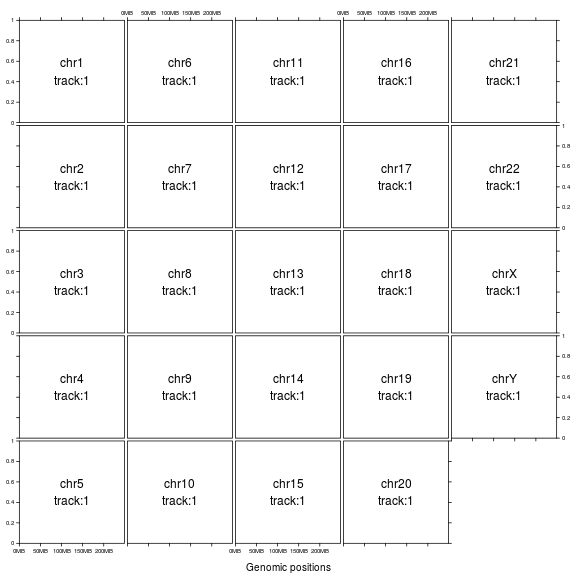Set gaps between chromosomes. Note if it is set as a numeric value, it should only be 0 (no gap).

``````gtrellis_layout(gap = 0)
``````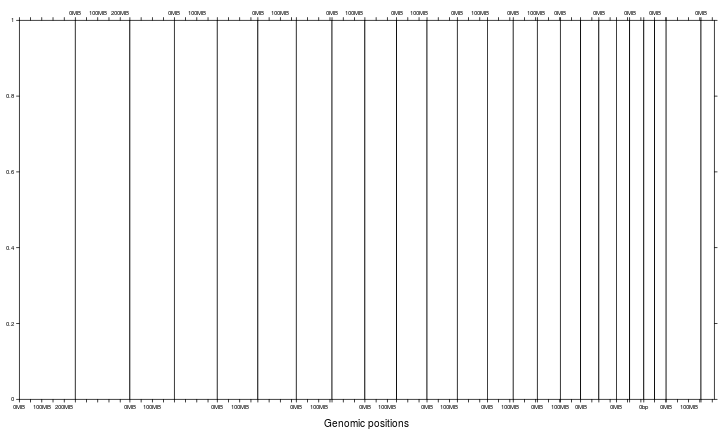Or `gap` can be a `unit` object.

``````gtrellis_layout(gap = unit(5, "mm"))
``````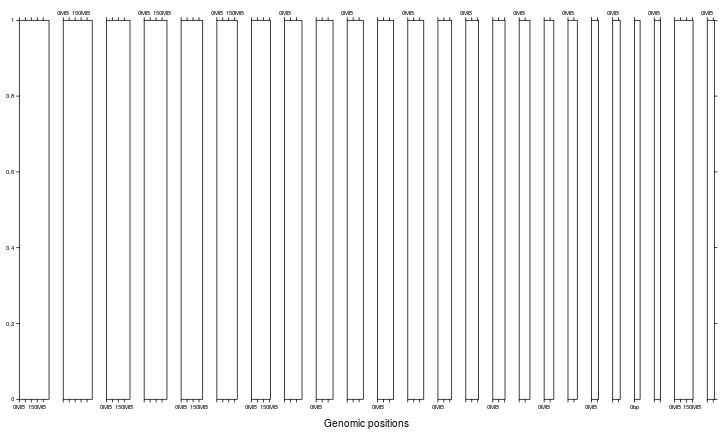When you arrange the layout with multiple rows, you can also set `gap` as length of two. In this case, the first element corresponds to the gaps between rows and the second corresponds to the gaps between columns.

``````gtrellis_layout(ncol = 5, gap = unit(c(5, 2), "mm"))
``````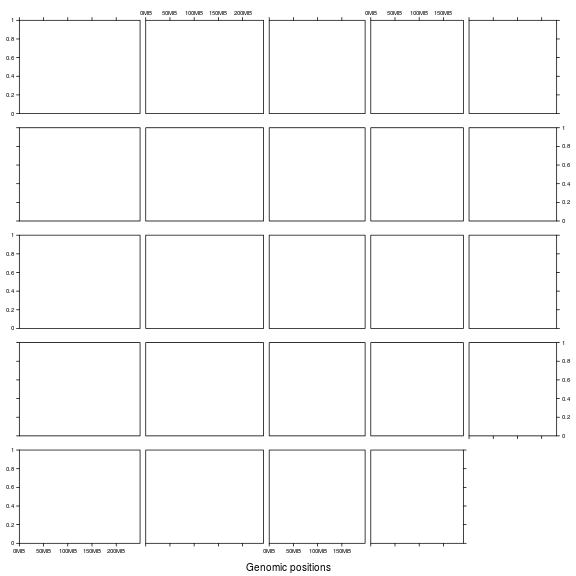There may be multiple tracks for chromosomes which describe multiple dimensional data. The tracks can be created by `n_track` argument.

``````gtrellis_layout(n_track = 3)
gtrellis_show_index()
``````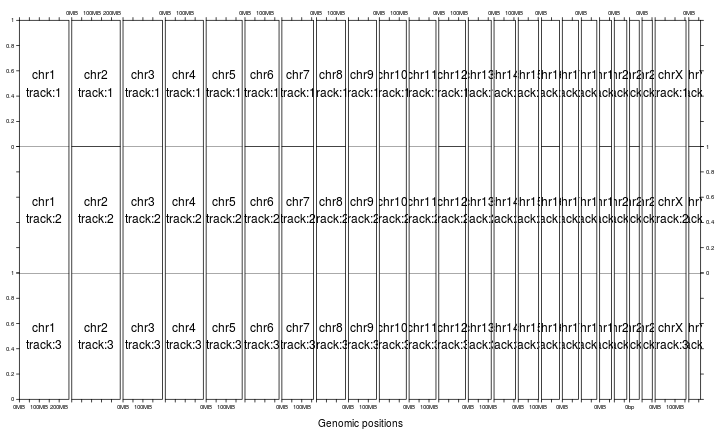By default, tracks share the same height. The height can be customized by `track_height` argument. If it is set as numeric values, it will be normalized as percent to the sum.

``````gtrellis_layout(n_track = 3, track_height = c(1, 2, 3))
``````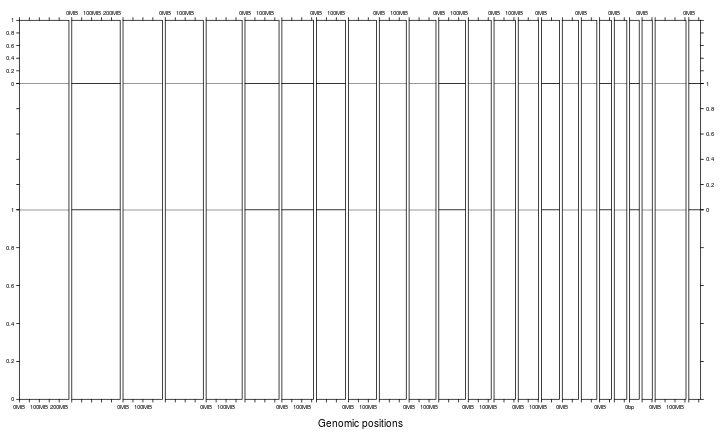`track_height` can also be a `unit` object.

``````gtrellis_layout(n_track = 3,
track_height = unit.c(unit(1, "cm"), unit(1, "null"), grobHeight(textGrob("chr1"))))
``````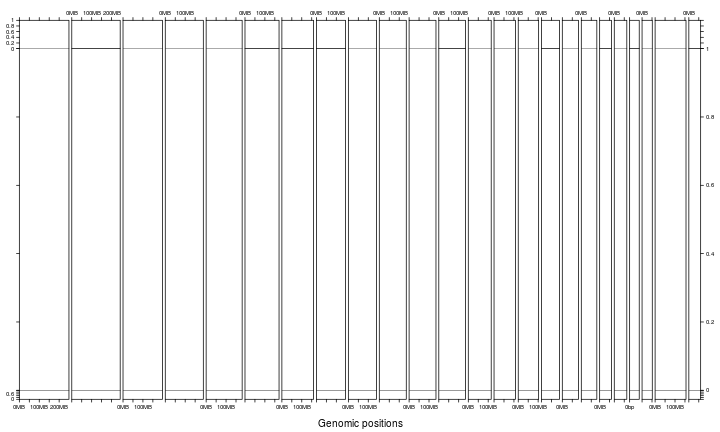Whether to show y-axes by setting `track_axis`. If certain value is set to `FALSE`, y-axis on corresponding track will not be drawn.

``````gtrellis_layout(n_track = 3, track_axis = c(FALSE, TRUE, FALSE), xaxis = FALSE, xlab = "")
``````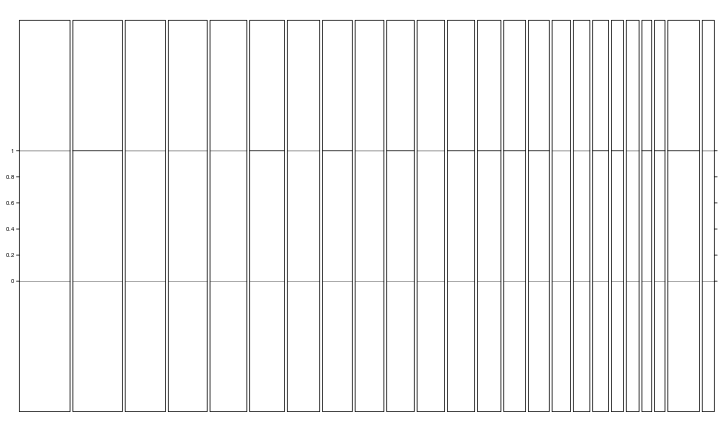Set y-lim by `track_ylim`. It should be a two-column matrix. But to make things easy, it can also be a vector and it will be filled into a two-column matrix by rows. If it is a vector with length 2, it means all tracks share the same y-lim.

``````gtrellis_layout(n_track = 3, track_ylim = c(0, 3, -4, 4, 0, 1000000))
``````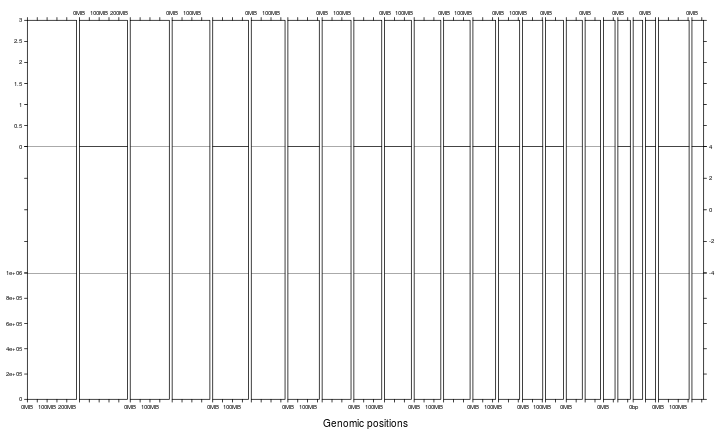Axis ticks are added on one side of rows or columns, `asist_ticks` controls whether to add axis ticks on the other sides. (You can compare following figure to the above one.)

``````gtrellis_layout(n_track = 3, track_ylim = c(0, 3, -4, 4, 0, 1000000), asist_ticks = FALSE)
``````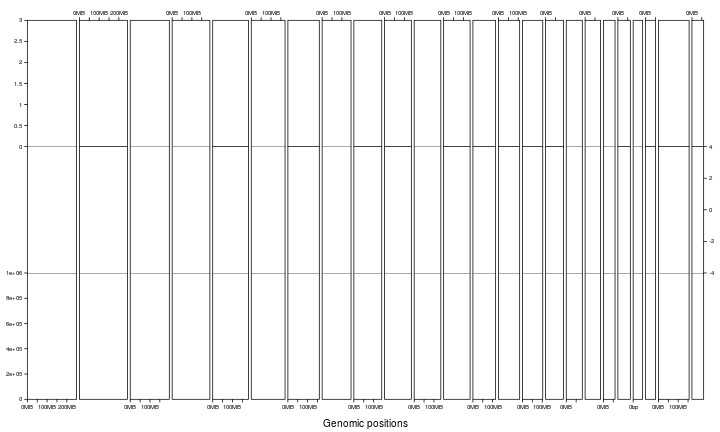Set x-label by `xlab` and set y-labels by `track_ylab`.

``````gtrellis_layout(n_track = 3, title = "title", track_ylab = c("", "bbbbbb", "ccccccc"), xlab = "xlab")
``````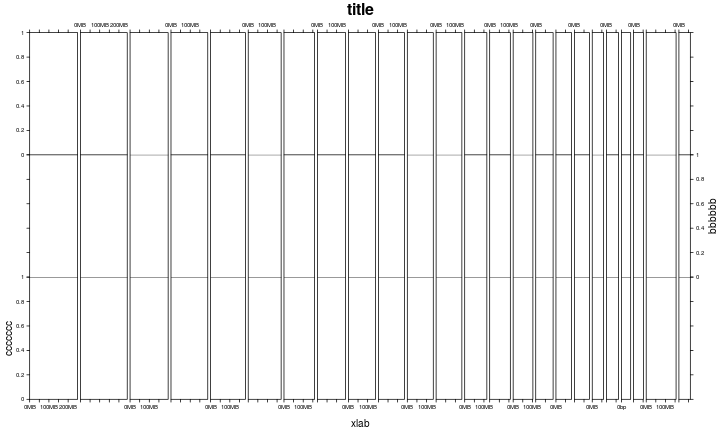Since chromosomes can have more than one tracks, following shows a layout with multiple columns and multiple tracks.

``````gtrellis_layout(n_track = 3, ncol = 4)
gtrellis_show_index()
``````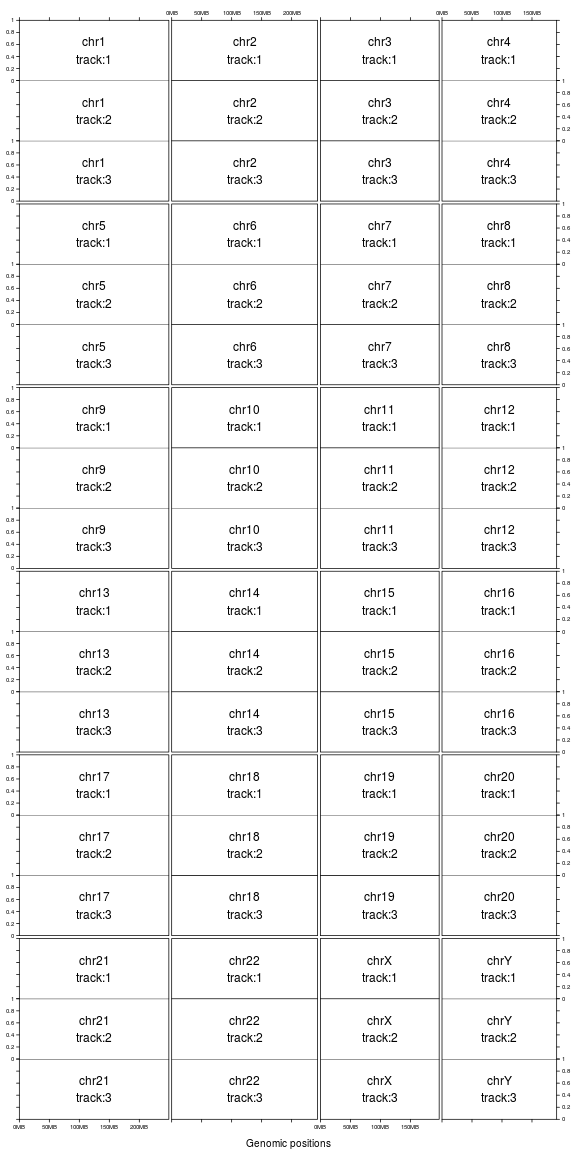Set `border` to `FALSE` to remove borders.

``````gtrellis_layout(n_track = 3, ncol = 4, border = FALSE, xaxis = FALSE, track_axis = FALSE, xlab = "")
gtrellis_show_index()
``````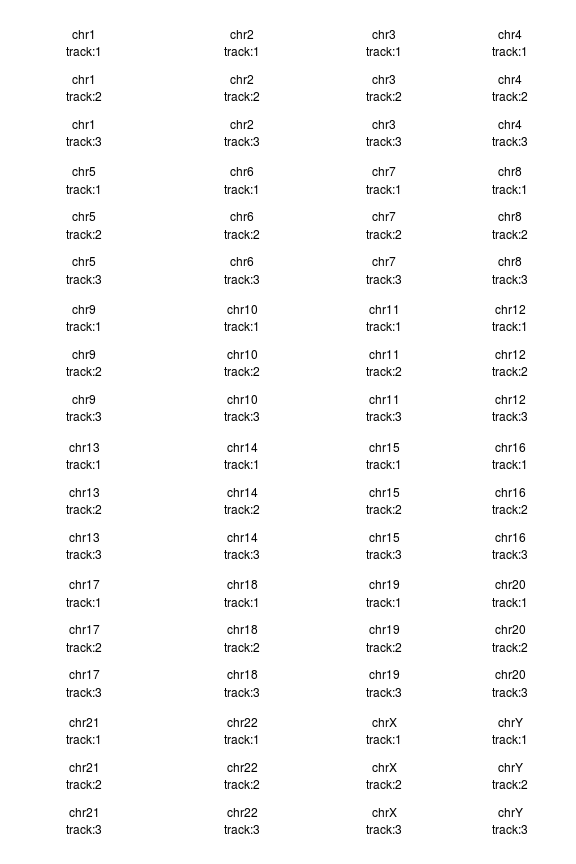## Add graphics track by track

After the initialization of the layout, each cell can be thought as an ordinary coordinate system. Then graphics can be added in afterwards.

Graphics are added by the self-defined function `panel.fun`. Similar as circlize package, `panel.fun` will be applied in every cell in 'the current track'. The first argument of `add_track()` can be either a `GRanges` object or a data frame, and the argument in `panel.fun` is a subset of data in current chromosome.

``````library(circlize)
bed = generateRandomBed()
gtrellis_layout(track_ylim = range(bed[]))
# `bed` inside `panel.fun` is a subset of the main `bed`
x = (bed[] + bed[]) / 2
y = bed[]
grid.points(x, y, pch = 16, size = unit(0.5, "mm"))
})
``````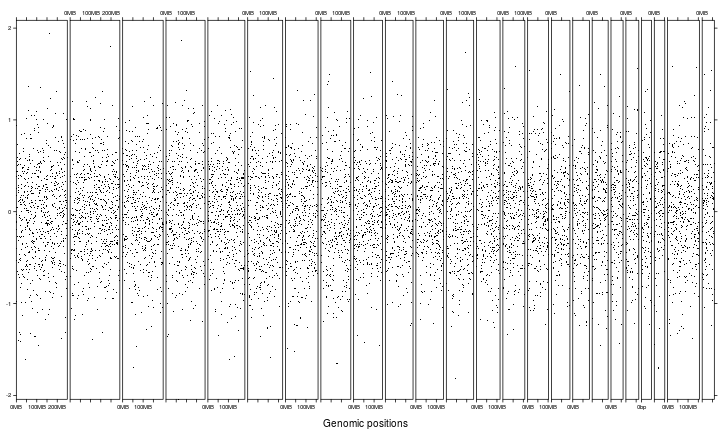The input data can be a `GRanges` object as well.

``````library(GenomicRanges)
gr = GRanges(seqnames = bed[],
ranges = IRanges(start = bed[],
end = bed[]),
score = bed[])
gtrellis_layout(track_ylim = range(gr\$score))
x = (start(gr) + end(gr)) / 2
y = gr\$score
grid.points(x, y, pch = 16, size = unit(0.5, "mm"))
})
``````Initialization and adding graphics are actually independent. Following example uses same code to add graphics but with different layout. (Compare to the first figure in this section.)

``````gtrellis_layout(nrow = 5, byrow = FALSE, track_ylim = range(bed[]))
x = (bed[] + bed[]) / 2
y = bed[]
grid.points(x, y, pch = 16, size = unit(0.5, "mm"))
})
``````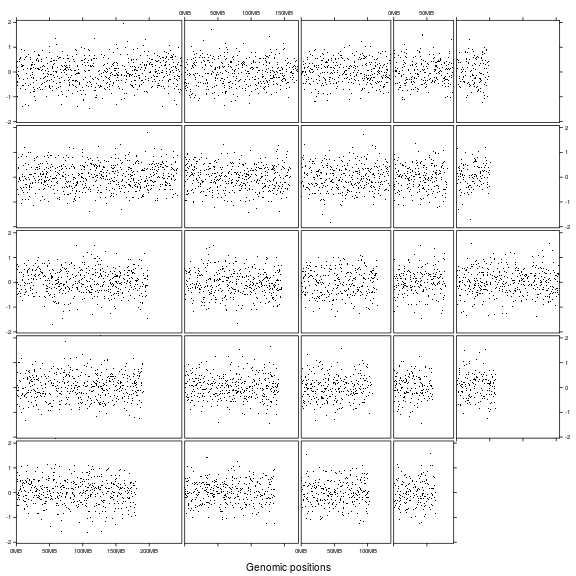In following, we make rainfall plot as well as the density distribution of genomic regions (in the example below, `DMR_hyper` contains differentially methylated regions that show high methylation compared to control samples and in `DMR_hypo` the methylation is lower than control samples). Also, we manually add a track which contains chromosome names and a track which contains ideograms.

Density for genomic regions is defined as the percent of a genomic window that is covered by the input genomic regions.

``````load(paste0(system.file("extdata", package = "circlize"), "/DMR.RData"))
DMR_hyper_density = lapply(split(DMR_hyper, DMR_hyper[]), function(gr) {
gr2 = circlize::genomicDensity(gr[2:3], window.size = 5e6)
cbind(chr = rep(gr[1,1], nrow(gr2)), gr2)
})
DMR_hyper_density = do.call("rbind", DMR_hyper_density)
``````
``````##         chr    start      end       pct
## chr1.1 chr1        1  5000000 0.0060636
## chr1.2 chr1  2500001  7500000 0.0036004
## chr1.3 chr1  5000001 10000000 0.0015556
## chr1.4 chr1  7500001 12500000 0.0024016
## chr1.5 chr1 10000001 15000000 0.0023680
## chr1.6 chr1 12500001 17500000 0.0027954
``````

Initialize the layout and add following four tracks:

1. chromosome names, simple text.
2. rainfall plot, first apply rainfall transformation and then add points.
3. genomic density, lines with area (actually it is polygons).
4. ideogram, rectangles.
``````gtrellis_layout(n_track = 4, ncol = 4, byrow = FALSE,
track_axis = c(FALSE, TRUE, TRUE, FALSE),
track_height = unit.c(1.5*grobHeight(textGrob("chr1")),
unit(1, "null"),
unit(0.5, "null"),
unit(3, "mm")),
track_ylim = c(0, 1, 0, 8, c(0, max(DMR_hyper_density[])), 0, 1),
track_ylab = c("", "log10(inter_dist)", "density", ""))

# track for chromosome names
chr = get_cell_meta_data("name")
grid.rect(gp = gpar(fill = "#EEEEEE"))
grid.text(chr)
})

# track for rainfall plots
df = circlize::rainfallTransform(gr[2:3])
x = (df[] + df[])/2
y = log10(df[])
grid.points(x, y, pch = 16, size = unit(0.5, "mm"), gp = gpar(col = "red"))
})

# track for genomic density
x = (gr[] + gr[])/2
y = gr[]
grid.polygon(c(x, x, x[length(x)]),
c(0, y, 0), default.units = "native", gp = gpar(fill = "pink"))
})

# track for ideogram
cytoband_chr = gr
grid.rect( cytoband_chr[], unit(0, "npc"),
width = cytoband_chr[] - cytoband_chr[], height = unit(1, "npc"),
default.units = "native", hjust = 0, vjust = 0,
gp = gpar(fill = circlize::cytoband.col(cytoband_chr[])) )
grid.rect( min(cytoband_chr[]), unit(0, "npc"),
width = max(cytoband_chr[]) - min(cytoband_chr[]), height = unit(1, "npc"),
default.units = "native", hjust = 0, vjust = 0,
gp = gpar(fill = "transparent") )
})
``````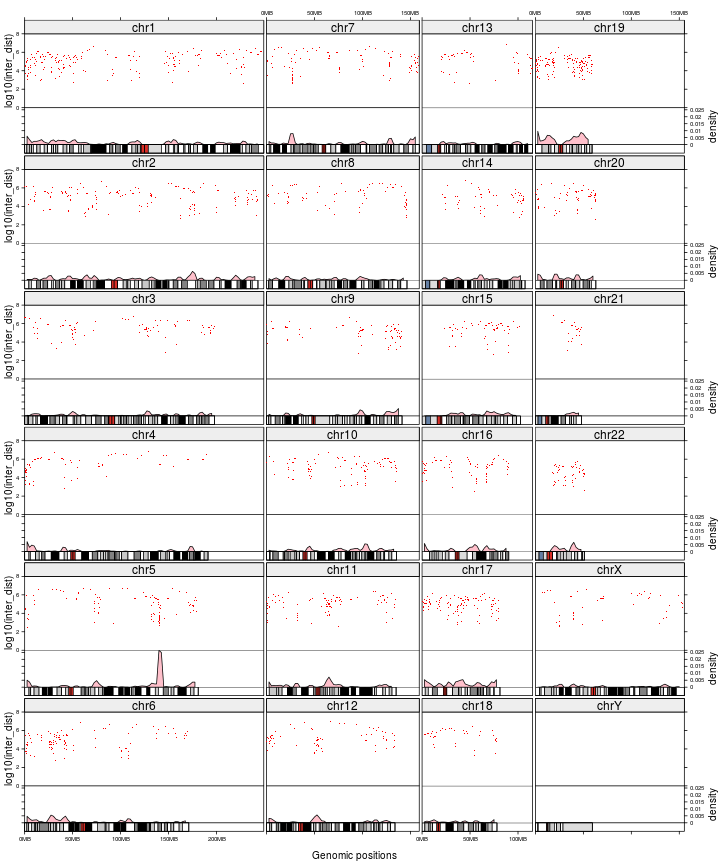Actually, you don't need to add name track and ideogram track manually. Name track and ideogram track can be added by `add_name_track` and `add_ideogram_track` arguments. Name track will be inserted before the first track and ideogram track will be inserted after the last track. So in following example, although we only specified `n_track` to 2, but the name track and ideogram track are also added, thus, the final number of track is 4.

In following example, we additionally add graphics for hypo-DMR as well so that direct comparison between different methylation patterns can be applied. Since rainfall plot for both hyper-DMR and hypo-DMR is added in a same track, we explicitly specify value of `track` argument to 2 in `add_track()`.

``````DMR_hypo_density = lapply(split(DMR_hypo, DMR_hypo[]), function(gr) {
gr2 = genomicDensity(gr[2:3], window.size = 5e6)
cbind(chr = rep(gr[1,1], nrow(gr2)), gr2)
})
DMR_hypo_density = do.call("rbind", DMR_hypo_density)

gtrellis_layout(n_track = 2, ncol = 4, byrow = FALSE,
track_axis = TRUE,
track_height = unit.c(unit(1, "null"),
unit(0.5, "null")),
track_ylim = c(0, 8, c(0, max(c(DMR_hyper_density[], DMR_hypo_density[])))),
track_ylab = c("log10(inter_dist)", "density"),
add_track(DMR_hyper, track = 2, panel.fun = function(gr) {
df = rainfallTransform(gr[2:3])
x = (df[] + df[])/2
y = log10(df[])
grid.points(x, y, pch = 16, size = unit(0.5, "mm"), gp = gpar(col = "#FF000040"))
})
add_track(DMR_hypo, track = 2, panel.fun = function(gr) {
df = rainfallTransform(gr[2:3])
x = (df[] + df[])/2
y = log10(df[])
grid.points(x, y, pch = 16, size = unit(0.5, "mm"), gp = gpar(col = "#0000FF40"))
})
add_track(DMR_hyper_density, track = 3, panel.fun = function(gr) {
x = (gr[] + gr[])/2
y = gr[]
grid.polygon(c(x, x, x[length(x)]),
c(0, y, 0), default.units = "native", gp = gpar(fill = "#FF000040"))
})
add_track(DMR_hypo_density, track = 3, panel.fun = function(gr) {
x = (gr[] + gr[])/2
y = gr[]
grid.polygon(c(x, x, x[length(x)]),
c(0, y, 0), default.units = "native", gp = gpar(fill = "#0000FF40"))
})
``````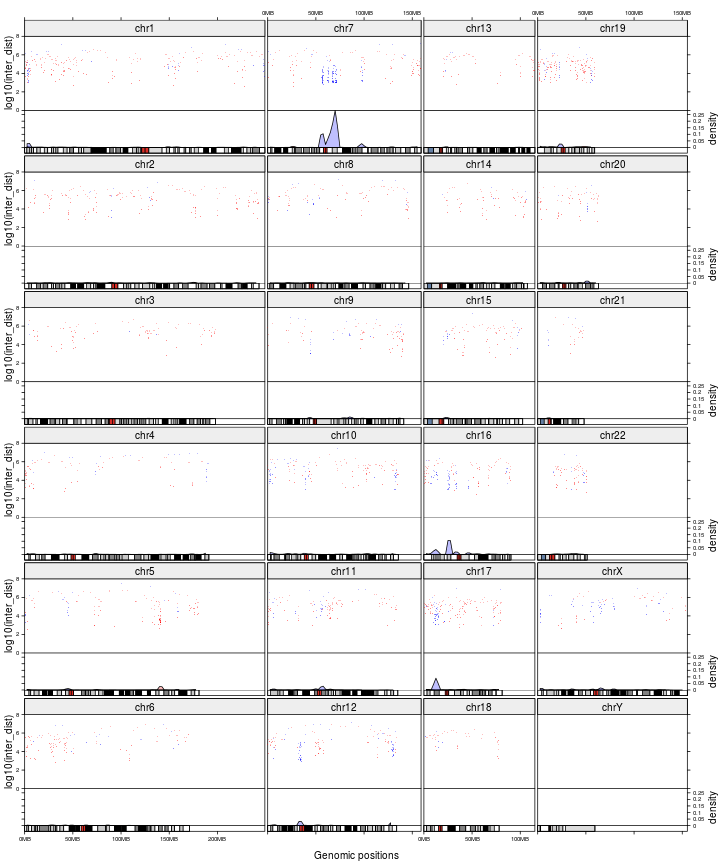By default, tracks are added from the first track to the last one. You can also add graphics in any specified chromosomes and tracks by specifying `category` and `track`.

``````all_chr = paste0("chr", 1:22)
letter = strsplit("MERRY CHRISTMAS!", "")[]
gtrellis_layout(nrow = 5)
for(i in seq_along(letter)) {
add_track(category = all_chr[i], track = 1, panel.fun = function(gr) {
grid.text(letter[i], gp = gpar(fontsize = 30))
})
}
``````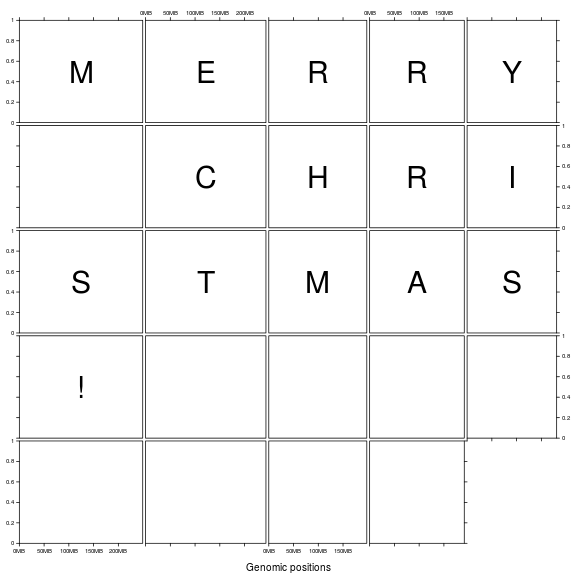Adding legend is not so straightforward, but it is not complex if you use functionality of the `grid` system. For example, you can first create a global viewport which contains a two-column layout, then put Trellis plot in one part and put legend in the other part. Remember to set `newpage` to `FALSE` so that Trellis plot will be added on the current graphic page.

``````legd = legendGrob("label", pch = 16)
layout = grid.layout(nrow = 1, ncol = 2, widths = unit.c(unit(1, "null"), grobWidth(legd)))
grid.newpage()
pushViewport(viewport(layout = layout))

pushViewport(viewport(layout.pos.row = 1, layout.pos.col = 1))
gtrellis_layout(nrow = 5, byrow = FALSE, track_ylim = range(bed[]), newpage = FALSE)
x = (bed[] + bed[]) / 2
y = bed[]
grid.points(x, y, pch = 16, size = unit(0.5, "mm"))
})
upViewport()

pushViewport(viewport(layout.pos.row = 1, layout.pos.col = 2))
grid.draw(legd)
upViewport()
``````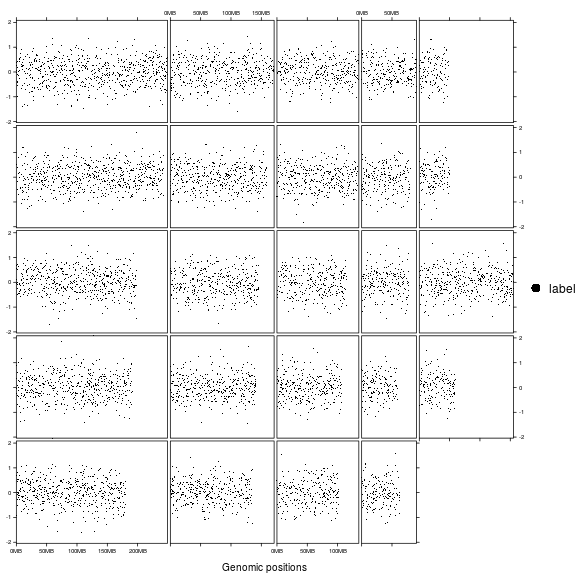As a real application, following code plots coverage for a tumor sample, its companion normal sample and the ratio of coverage. First prepare the data:

``````tumor_df = readRDS(paste0(system.file("extdata", package = "gtrellis"), "/df_tumor.rds"))
control_df = readRDS(paste0(system.file("extdata", package = "gtrellis"), "/df_control.rds"))

# remove regions that have zero coverage
ind = which(tumor_df\$cov > 0 & control_df\$cov > 0)
tumor_df = tumor_df[ind, , drop = FALSE]
control_df = control_df[ind, , drop = FALSE]
ratio_df = tumor_df

# get rid of small value dividing small value resulting large value
q01 = quantile(c(tumor_df\$cov, control_df\$cov), 0.01)
ratio_df[] = log2( (tumor_df\$cov+q01) / (control_df\$cov+q01) *
sum(control_df\$cov) / sum(tumor_df\$cov) )
names(ratio_df) = c("chr", "start", "end", "ratio")
tumor_df[] = log10(tumor_df[])
control_df[] = log10(control_df[])
``````

Then, initialize the layout and add three tracks.

``````cov_range = range(c(tumor_df[], control_df[]))
ratio_range = range(ratio_df[])
ratio_range = c(-max(abs(ratio_range)), max(abs(ratio_range)))

gtrellis_layout(n_track = 3, nrow = 3, byrow = FALSE, gap = unit(c(4, 1), "mm"),
track_ylim = c(cov_range, cov_range, ratio_range),
track_ylab = c("tumor, log10(cov)", "control, log10(cov)", "ratio, log2(ratio)"),

# track for coverage in tumor
x = (gr[] + gr[])/2
y = gr[]
grid.points(x, y, pch = 16, size = unit(2, "bigpts"), gp = gpar(col = "#00000020"))
})

# track for coverage in control
x = (gr[] + gr[])/2
y = gr[]
grid.points(x, y, pch = 16, size = unit(2, "bigpts"), gp = gpar(col = "#00000020"))
})

# track for ratio between tumor and control
library(RColorBrewer)
col_fun = circlize::colorRamp2(seq(-0.5, 0.5, length = 11), rev(brewer.pal(11, "RdYlBu")),
transparency = 0.5)
x = (gr[] + gr[])/2
y = gr[]
grid.points(x, y, pch = 16, size = unit(2, "bigpts"), gp = gpar(col = col_fun(y)))
grid.lines(unit(c(0, 1), "npc"), unit(c(0, 0), "native"), gp = gpar(col = "#0000FF80"))
})
``````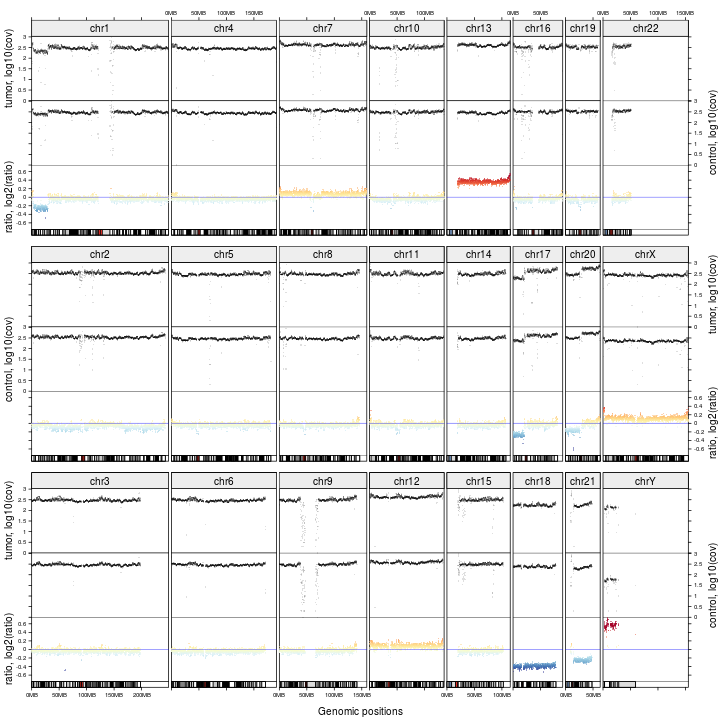Following example visualizes gene density (defined as how much a genomic window is covered by gene regions) on different chromosomes both by a line track and a heatmap track.

``````gene = readRDS(paste0(system.file(package = "gtrellis"),
"/extdata/gencode_v19_protein_coding_genes.rds"))
gene_density = lapply(split(gene, gene[]), function(gr) {
gr2 = genomicDensity(gr[2:3], window.size = 5e6)
cbind(chr = rep(gr[1,1], nrow(gr2)), gr2)
})
gene_density = do.call("rbind", gene_density)

gtrellis_layout(byrow = FALSE, n_track = 2, ncol = 4,
track_ylim = c(0, max(gene_density[]), 0, 1), track_axis = c(TRUE, FALSE),
track_height = unit.c(unit(1, "null"), unit(4, "mm")),
track_ylab = c("density", ""))

x = (gr[] + gr[])/2
y = gr[]
grid.lines(x, y, default.unit = "native")
})

col_fun = circlize::colorRamp2(seq(0, max(gene_density[]), length = 11),
rev(brewer.pal(11, "RdYlBu")))
grid.rect(gr[], 0, width = gr[] - gr[], height = 1, just = c(0, 0),
default.units = "native", gp = gpar(fill = col_fun(gr[]), col = NA))
})
``````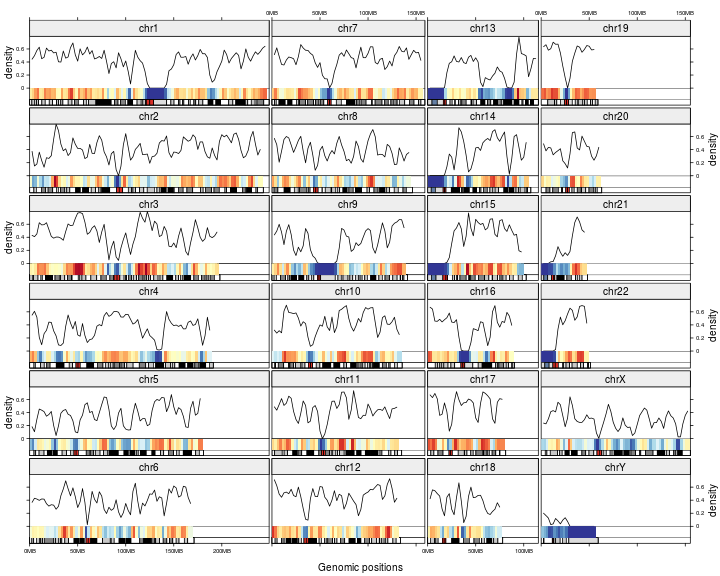Following figure is karyogram view of genomic regions (reproduced from http://www.tengfei.name/ggbio/docs/man/layout_karyogram-method.html). We arrange the layout as one column and create two 'short' tracks, one for genomic regions and one for ideogram. Different values are mapped to continuous colors.

We specified `n_track` to 1, but we also specify `add_ideogram_track` to `TRUE`, so actually there are two tracks. `xpadding` is specified to make some space on the left of the cells so that We can manually add chromosome names in the second track.

``````bed = generateRandomBed(nr = 10000)
bed = bed[sample(10000, 100), ]
col_fun = colorRamp2(c(-1, 0, 1), c("green", "yellow", "red"))

gtrellis_layout(n_track = 1, ncol = 1, track_axis = FALSE, xpadding = c(0.1, 0),
gap = unit(4, "mm"), border = FALSE, asist_ticks = FALSE, add_ideogram_track = TRUE,
ideogram_track_height = unit(2, "mm"))
grid.rect((gr[] + gr[])/2, unit(0.2, "npc"), unit(1, "mm"), unit(0.8, "npc"),
hjust = 0, vjust = 0, default.units = "native",
gp = gpar(fill = col_fun(gr[]), col = NA))
})
add_track(track = 2, clip = FALSE, panel.fun = function(gr) {
chr = get_cell_meta_data("name")
if(chr == "chrY") {
grid.lines(get_cell_meta_data("xlim"), unit(c(0, 0), "npc"),
default.units = "native")
}
grid.text(chr, x = 0, y = 0, just = c("left", "bottom"))
})

breaks = seq(-1, 1, by = 0.5)
lg = legendGrob(breaks, pch = 15, vgap = 0, gp = gpar(col = col_fun(breaks)))
pushViewport(viewport(0.9, 0.1, width = grobWidth(lg), height = grobHeight(lg), just = c(1, 0)))
grid.draw(lg)
upViewport()
``````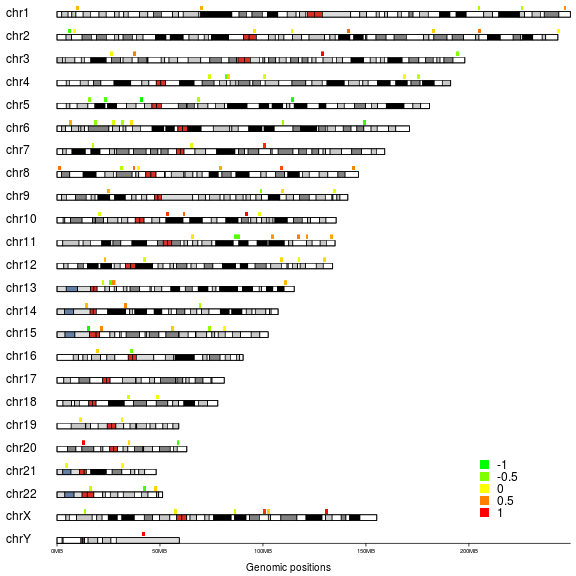## Get meta data for each cell

For every cell in the plot, several meta data can be extracted by `get_cell_meta_data()`. `get_cell_meta_data()` is always used inside `panel.fun` to extract information about the 'current cell'. You can also use the function outside `panel.fun` by explicitly specifying `category` and `track`. Pseudo code are:

``````gtrellis_layout()
# get xlim of current cell
xlim = get_cell_meta_data("xlim")
})

# get xlim of the specified cell
xlim = get_cell_meta_data("xlim", category = "chr2", track = 1)
``````

Following meta data can be retrieved by `get_cell_meta_data()`:

• `name`: category name.
• `xlim`: xlim without including padding, cells in a same column shares the same `xlim`.
• `ylim`: ylim without including padding.
• `extended_xlim`: xlim with padding.
• `extended_ylim`: ylim with padding.
• `original_xlim`: xlim in original data.
• `original_ylim`: ylim in original data.
• `column`: which column in the layout.
• `row`: which row in the layout.
• `track`: which track in the layout.

Following figure demonstrates difference between different cell meta data. The space between `extended_xlim` and `xlim` is the padding regions on x direction.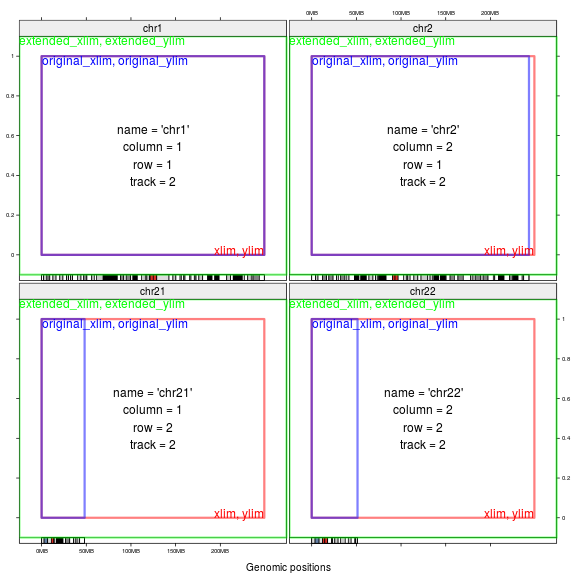## General genomic categories

Genomic categories are not restricted in chromosomes. It can be any kind, such as genes. Similar as `circlize::circos.genomicInitialize()`, you can also specify genomic categories as well as their ranges as a data frame when initializing the layout.

In following example, we put three genes in one row and add their transcripts afterwards.

``````load(paste0(system.file(package = "circlize"), "/extdata/tp_family.RData"))
df = data.frame(gene = names(tp_family),
start = sapply(tp_family, function(x) min(unlist(x))),
end = sapply(tp_family, function(x) max(unlist(x))))
df
``````
``````##      gene     start       end
## TP73 TP73   3569084   3652765
## TP63 TP63 189349205 189615068
## TP53 TP53   7565097   7590856
``````
``````# maximum number of transcripts
n = max(sapply(tp_family, length))

gtrellis_layout(data = df, n_track = 1, track_ylim = c(0.5, n+0.5),
gn = get_cell_meta_data("name")
tr = tp_family[[gn]] # all transcripts for this gene
for(i in seq_along(tr)) {
# for each transcript
current_tr_start = min(tr[[i]]\$start)
current_tr_end = max(tr[[i]]\$end)
grid.lines(c(current_tr_start, current_tr_end), c(n - i + 1, n - i + 1),
default.units = "native", gp = gpar(col = "#CCCCCC"))
grid.rect(tr[[i]][], n - i + 1, tr[[i]][] - tr[[i]][], 0.8,
default.units = "native", just = "left",
gp = gpar(fill = "orange", col = "orange"))
}
})
``````